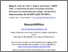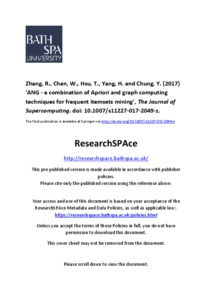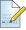# ANG - a combination of Apriori and graph computing techniques for frequent itemsets mining

Zhang, R, Chen, W, Hsu, T, Yang, H and Chung, Y (2017) 'ANG - a combination of Apriori and graph computing techniques for frequent itemsets mining.' The Journal of Supercomputing. ISSN 1573-0484Preview
Text
9490.pdf - Accepted Version
Repository Terms Apply.

Download (890kB) | Preview
Official URL: http://dx.doi.org/10.1007/s11227-017-2049-z

## Abstract

The Apriori algorithm is one of the most well-known and widely accepted methods for the association rule mining. In Apriori, it uses a prefix tree to represent k-itemsets, generates k-itemset candidates based on the frequent (k−1)-itemsets, and determines the frequent k-itemsets by traversing the prefix tree iteratively based on the transaction records. When k is small, the execution of Apriori is very efficient. However, the execution of Apriori could be very slow when k becomes large because of the deeper recursion depth to determine the frequent k-itemsets. From the perspective of graph computing, the transaction records can be converted to a graph G(V,E), where V is the set of vertices of G that represents the transaction records and E is the set of edges of G that represents the relations among transaction records. Each k-itemset in the transaction records will have a corresponding connected component in G. The number of vertices in the corresponding connected component is the support of the k-itemset. Since the time to find the corresponding connected component of a k-itemset in G is constant for any k, the graph computing method will be very efficient if the number of k-itemsets is relatively small. Based on Apriori and graph computing techniques, a hybrid method, called Apriori and Graph Computing (ANG), is proposed to compute the frequent itemsets. Initially, ANG uses Apriori to compute the frequent k-itemsets and then switches to the graph computing method when k becomes large (where the number of k-itemset candidates is relatively small). The experimental results show that ANG outperforms both Apriori and the graph computing method for all test cases.

Item Type: Article Apriori, graph computing, frequent itemset mining, data mining College of Liberal Arts 19 Apr 2017 14:44 22 May 2019 08:00Request a change to this item or report an issueUpdate item (repository staff only)